# Selina Solutions Concise Maths Class 7 Chapter 18: Recognition of Solids (Representing 3-D in 2-D)

Selina Solutions Concise Maths Class 7 Chapter 18 Recognition of Solids (Representing 3-D in 2-D) provides basic knowledge among students, as per the ICSE syllabus. The solutions created mainly boost confidence among students in solving tricky problems. It also improves their time management skills to face the annual exam without fear. Students can download Selina Solutions Concise Maths Class 7 Chapter 18 Recognition of Solids (Representing 3-D in 2-D) PDF, from the links which are provided below.

Chapter 18 covers important concepts like identification of 3D shapes, Euler’s formula, nets and mapping space around us. Students can use the solutions PDF to understand the method of solving problems effortlessly.

## Selina Solutions Concise Maths Class 7 Chapter 18: Recognition of Solids (Representing 3-D in 2-D) Download PDF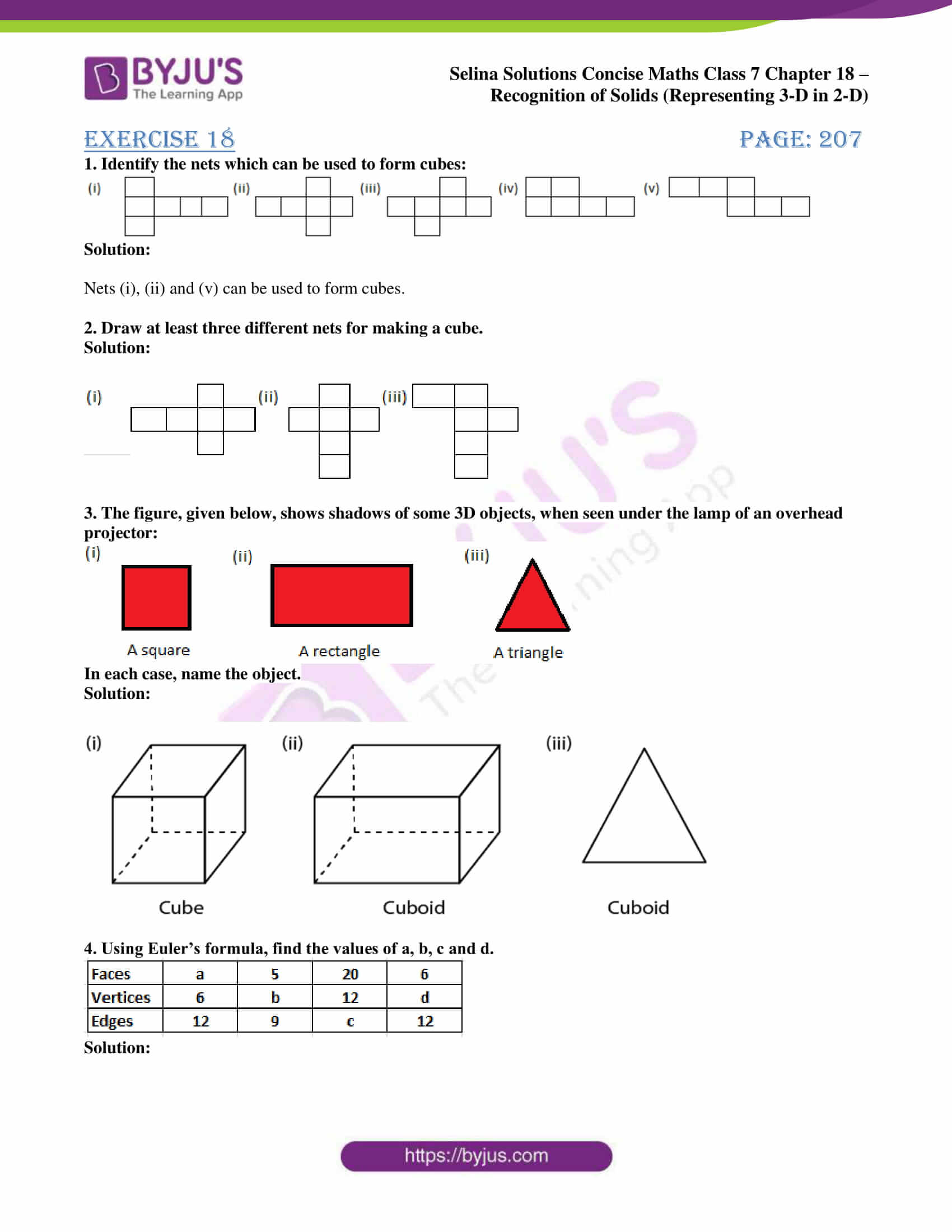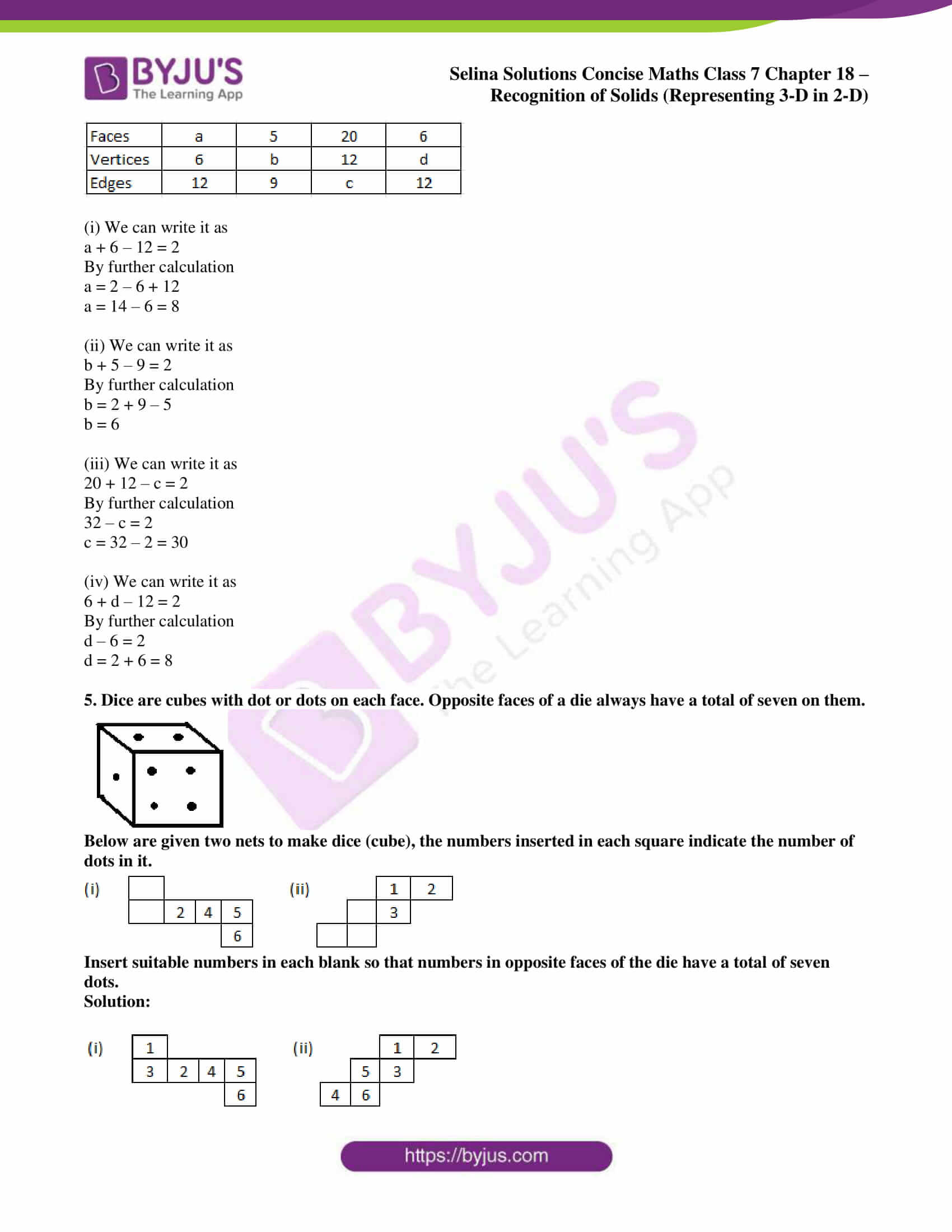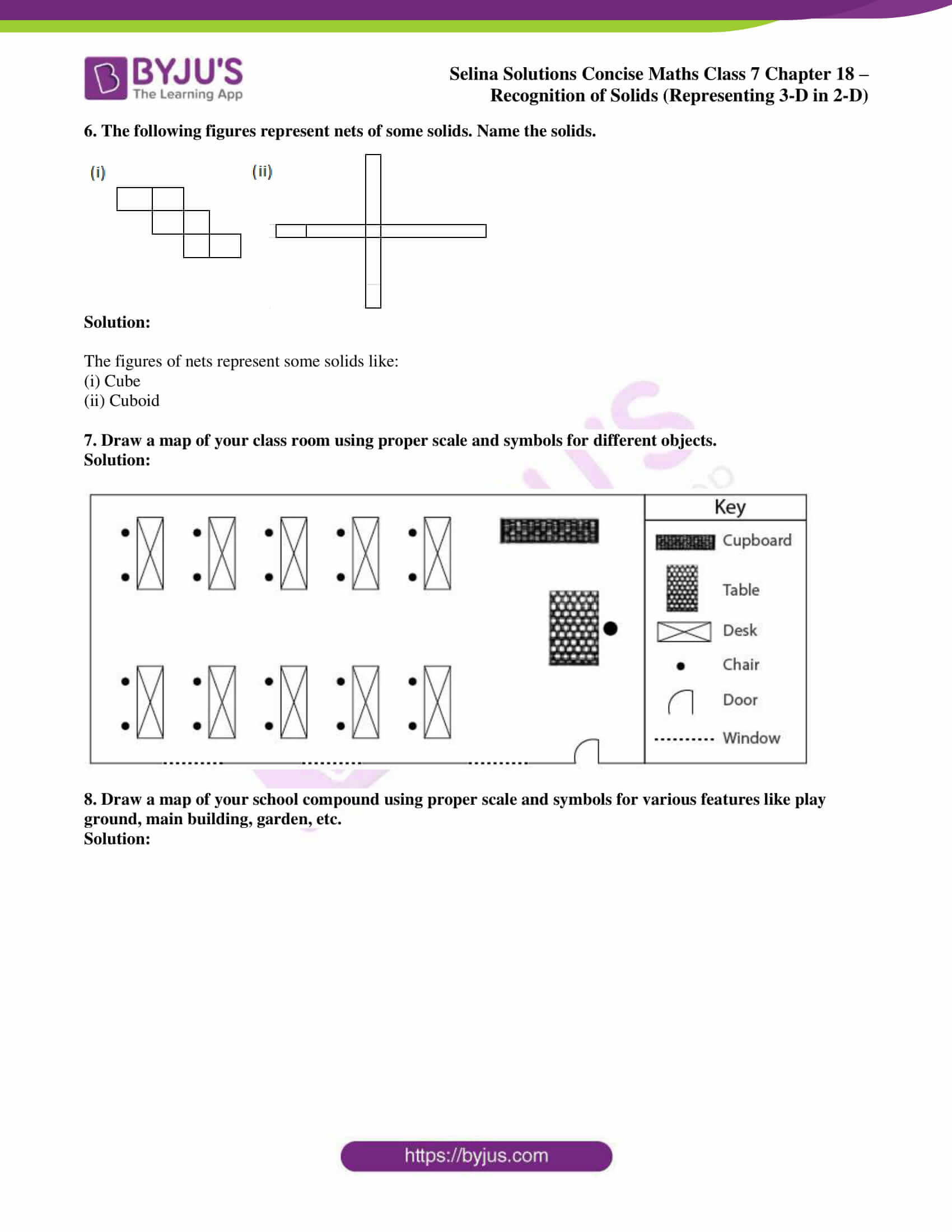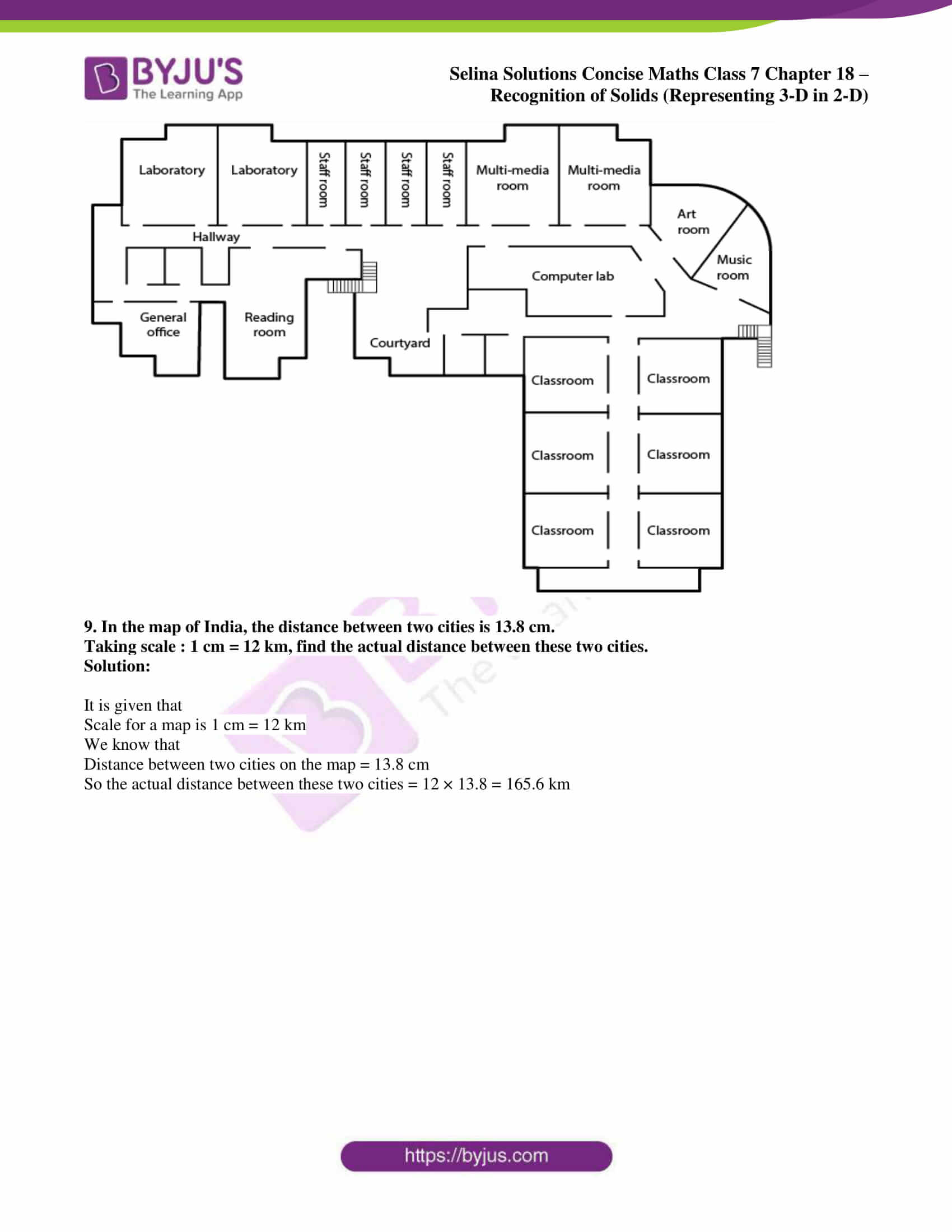## Access Selina Solutions Concise Maths Class 7 Chapter 18: Recognition of Solids (Representing 3-D in 2-D)

Exercise 18 page: 207

1. Identify the nets which can be used to form cubes: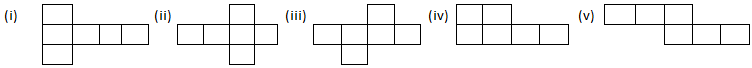Solution:

Nets (i), (ii) and (v) can be used to form cubes.

2. Draw at least three different nets for making a cube.

Solution: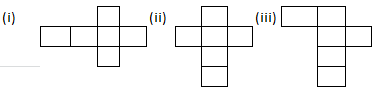3. The figure, given below, shows shadows of some 3D objects, when seen under the lamp of an overhead projector: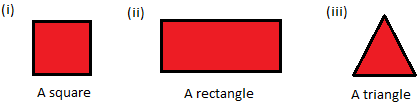In each case, name the object.

Solution: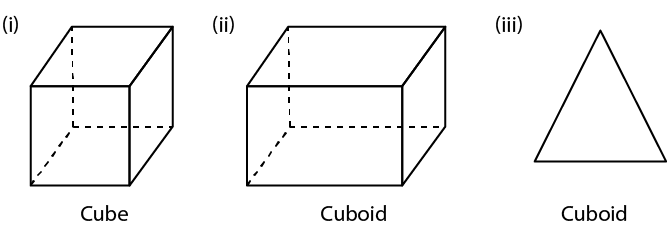4. Using Euler’s formula, find the values of a, b, c and d.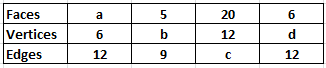Solution: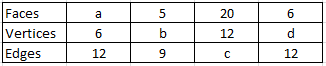(i) We can write it as

a + 6 – 12 = 2

By further calculation

a = 2 – 6 + 12

a = 14 – 6 = 8

(ii) We can write it as

b + 5 – 9 = 2

By further calculation

b = 2 + 9 – 5

b = 6

(iii) We can write it as

20 + 12 – c = 2

By further calculation

32 – c = 2

c = 32 – 2 = 30

(iv) We can write it as

6 + d – 12 = 2

By further calculation

d – 6 = 2

d = 2 + 6 = 8

5. Dice are cubes with dot or dots on each face. Opposite faces of a die always have a total of seven on them.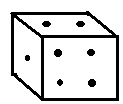Below are given two nets to make dice (cube), the numbers inserted in each square indicate the number of dots in it.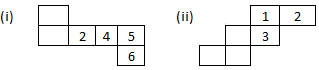Insert suitable numbers in each blank so that numbers in opposite faces of the die have a total of seven dots.

Solution: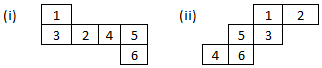6. The following figures represent nets of some solids. Name the solids.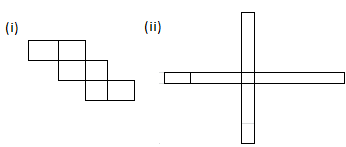Solution:

The figures of nets represent some solids like:

(i) Cube

(ii) Cuboid

7. Draw a map of your class room using proper scale and symbols for different objects.

Solution: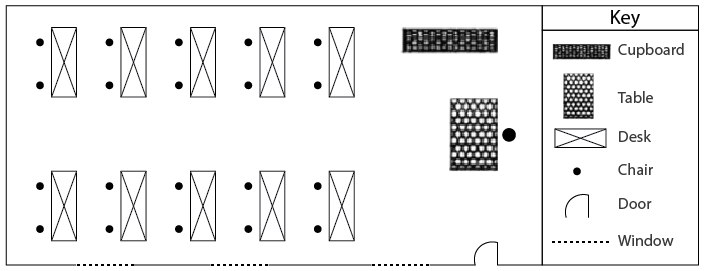8. Draw a map of your school compound using proper scale and symbols for various features like play ground, main building, garden, etc.

Solution: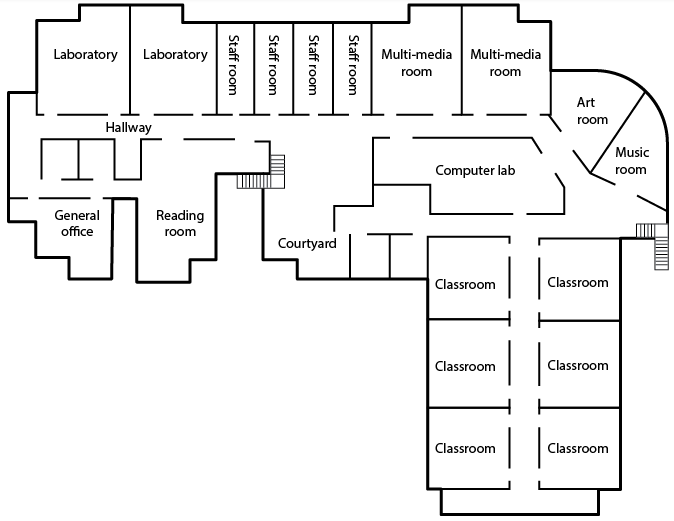9. In the map of India, the distance between two cities is 13.8 cm.

Taking scale : 1 cm = 12 km, find the actual distance between these two cities.

Solution:

It is given that

Scale for a map is 1 cm = 12 km

We know that

Distance between two cities on the map = 13.8 cm

So the actual distance between these two cities = 12 × 13.8 = 165.6 km